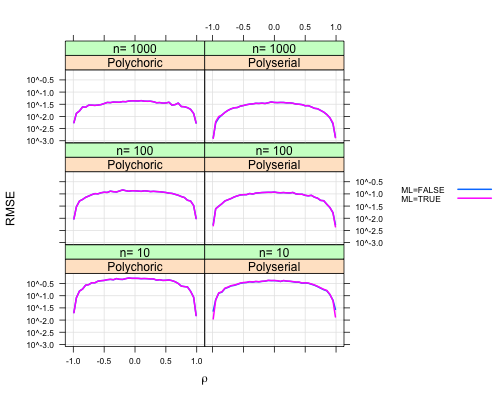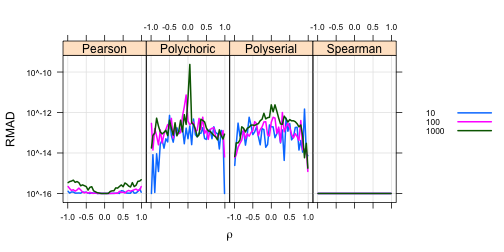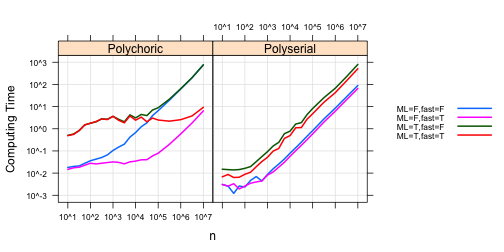The wCorr package can be used to calculate Pearson, Spearman, polyserial, and polychoric correlations, in weighted or unweighted form.[The estimation procedure used by the wCorr package for the polyserial is based on the likelihood function in by Cox, N. R. (1974), “Estimation of the Correlation between a Continuous and a Discrete Variable.” Biometrics, 30 (1), pp 171-178. The likelihood function for polychoric is from Olsson, U. (1979) “Maximum Likelihood Estimation of the Polychoric Correlation Coefficient.” Psyhometrika, 44 (4), pp 443-460. The likelihood used for Pearson and Spearman is written down many places. One is the “correlate” function in Stata Corp, Stata Statistical Software: Release 8. College Station, TX: Stata Corp LP, 2003.] The package implements the tetrachoric correlation as a specific case of the polychoric correlation and biserial correlation as a specific case of the polyserial correlation. When weights are used, the correlation coefficients are calculated with so called sample weights or inverse probability weights.[Sample weights are comparable to pweight in Stata.]

This vignette describes the use of applying two Boolean switches in the wCorr package. It describes the implications and uses simulation to show the impact of these switches on resulting correlation estimates.

First, the Maximum Likelihood, or ML switch uses the Maximum Likelihood Estimator (MLE) when ML=TRUE or uses a consistent but non-MLE estimator for the nuisance parameters when ML=FALSE. The simulations show that using ML=FALSE is preferable because it speeds computation and decreases the root mean square error (RMSE) of the estimator.

Second the fast argument gives the option to use a pure R implementation (fast=FALSE) or an implementation that relies on the Rcpp and RcppArmadillo packages (fast=TRUE). The simulations show agreement to within $$10^{-9}$$, showing the implementations agree. At the same time the fast=TRUE option is always as fast or faster.

In addition to this vignette, the wCorr Formulas vignette describes the statistical properties of the correlation estimators in the package and has a more complete derivation of the likelihood functions.

# The ML switch

The wCorr package computes correlation coefficients between two vectors of random variables that are jointly bivariate normal. We call the two vectors X and Y.

$\begin{pmatrix} X \ Y \end{pmatrix} \sim N \left[ \begin{pmatrix} \mu_x \ \mu_y \end{pmatrix}, \boldsymbol{\Sigma} \right]$

where $$N(\mathbf{A},\boldsymbol{\Sigma})$$ is the bivariate normal distribution with mean A and covariance $$\boldsymbol{\Sigma}$$.

## Computation of polyserial correlation

The likelihood function for an individual observation of the polyserial correlation is[See the wCorr Formulas vignette for a more complete description of the polyserial correlations' likelihood function.]

$\mathrm{Pr}\left( \rho=r, \boldsymbol{\Theta}=\boldsymbol{\theta} ; Z=z_i, M=m_i \right) = \phi(z_i) \left[ \Phi\left( \frac{\theta_{m_i+2} - r \cdot z_i}{\sqrt{1-r^2}} \right) - \Phi \left( \frac{\theta_{m_i+1} - r \cdot z_i}{\sqrt{1-r^2}} \right) \right]$

where $$\rho$$ is the correlation between X and Y, Z is the normalized version of X, and M is a discretized version of Y, using $$\boldsymbol{\theta}$$ as cut points as described in the wCorr Formulas vignette. Here an i is used to index the observed units.

The log-likelihood function ($$\ell$$) is then

$\ell(\rho, \boldsymbol{\Theta}=\boldsymbol{\theta};\mathbf{Z}=\mathbf{z},\mathbf{M}=\mathbf{m}) = \sum_{i=1}^n w_i \ln\left[ \mathrm{Pr}\left( \rho=r, \boldsymbol{\Theta}=\boldsymbol{\theta} ; Z=z_i, M=m_i \right) \right]$

The derivatives of $$\ell$$ can be written down but are not readily computed. When the ML argument is set to FALSE (the default), the values of $$\boldsymbol{\theta}$$ are computed using a consistent estimator[The value of the nuisance parameter $$\boldsymbol{\theta}$$ is chosen to be $$\Phi^{-1}(n/N)$$ where $$n$$ is the number of values to the left of the cut point ($$\theta_i$$ value) and $$N$$ is the number of data points overall. For the weighted cause $$n$$ is replaced by the sum of the weights to the left of the cut point and $$N$$ is replaced by the total weight of all units. See the wCorr Formulas vignette for a more complete description.] and a one dimensional optimization of $$\rho$$ is calculated using the optimize function in the stats package. When the ML argument is set to TRUE, a multi-dimensional optimization is done for $$\rho$$ and $$\boldsymbol{\theta}$$ using the bobyqa function in the minqa package.

## Computation of polychoric correlation

For the polychoric correlation the observed data is expressed in ordinal form for both variables. Here the discretized version of X is P and the discretized version of Y remains M.[See the “wCorr Formulas” vignette for a more complete description of the polychoric correlations' likelihood function.] The likelihood function for the polychoric is

$\mathrm{Pr}\left( \rho=r, \boldsymbol{\Theta}=\boldsymbol{\theta}, \boldsymbol{\Theta}‘=\boldsymbol{\theta}’ ; P=p_i, M=m_i \right) = \int_{\theta_{p_i+1}‘}^{\theta_{p_i+2}’} \int_{\theta_{m_i+1}}^{\theta_{m_i+2}} \mkern-40mu f(x,y|\rho=r) dy dx$

where $$f(x,y|r)$$ is the normalized bivariate normal distribution with correlation $$\rho$$, $$\boldsymbol{\theta}$$ are the cut points used to discretize Y into M, and $$\boldsymbol{\theta'}$$ are the cut points used to discretize X into P.

The log-likelihood is then $\ell(\rho, \boldsymbol{\Theta}=\boldsymbol{\theta}, \boldsymbol{\Theta}‘=\boldsymbol{\theta}’ ;\mathbf{P}=\mathbf{p}, \mathbf{M}=\mathbf{m}) = \sum_{i=1}^n w_i \ln\left[\mathrm{Pr}\left( \rho=r, \boldsymbol{\Theta}=\boldsymbol{\theta},\boldsymbol{\Theta}‘= \boldsymbol{\theta}’ ; P=p_i, M=m_i \right) \right]$

The derivatives of $$\ell$$ can be written down but are not readily computed. When the ML argument is set to FALSE (the default), the values of $$\boldsymbol{\theta}$$ and $$\boldsymbol{\theta}‘$$ are computed using a consistent estimator and a one dimensional optimization of $$\rho$$ is calculated using the optimize function in the stats package. When the ML argument is set to TRUE, a multi-dimensional optimization is done for $$\rho$$, $$\boldsymbol{\theta}$$, $$\boldsymbol{\theta}’$$ using the bobyqa function in the minqa package.

# Simulation study

To demonstrate the effect of the ML and fast switches a few simulation studies are performed to compare the similarity of the results when the switch is set to TRUE to the result when the switch is set to FALSE. This is done first for the ML switch and then for the fast switch.

Finally, simulations show the implications of these switches on the speed of the computation.

# General procedures of the simulation study of unweighted correlations

A simulation is run several times.[The exact number is noted for each specific simulation.] For each iteration, the following procedure is used:[When the exact method of selecting a parameter (such as $$n$$) is not noted above, it is described as part of each simulation.]

• select a true correlation coefficient $$\rho$$;
• select the number of observations $$n$$;
• generate X and Y to be bivariate normally distributed using a pseudo-Random Number Generator (RNG);
• using a pseudo-RNG, select the number of bins for M and P ($$t$$ and $$t'$$) independently from the set {2, 3, 4, 5};
• select the bin boundaries for M and P ($$\boldsymbol{\theta}$$ and $$\boldsymbol{\theta}‘$$) by sorting the results of $$(t-1)$$ and $$(t’-1)$$ draws, respectively, from a normal distribution using a pseudo-RNG;
• confirm that at least 2 levels of each of M and P are occupied (if not, return to the previous step); and
• calculate and record the correlation coefficients.

One of a few possible statistics is then calculated. To compare two levels of a switch the Relative Mean Absolute Deviation is used

$RMAD= \frac{1}{m} \sum_{j=1}^m | r_{j, \mathtt{TRUE}} - r_{j, \mathtt{FALSE}} |$

where there are $$m$$ simulations run, $$r_{j, \mathtt{TRUE}}$$ and $$r_{j, \mathtt{FALSE}}$$ are the estimated correlation coefficient for the $j$th simulated dataset when the switch is set to TRUE and FALSE, respectively. This statistic is called “relative” because it is compared to the other method of computing the statistic, not the true value.

To compare either level to the true correlation coefficient the Root Mean Square Error is used

$RMSE= \sqrt{ \frac{1}{m} \sum_{j=1}^m (r_j - \rho_j)^2 }$

where, for the $j$th simulated dataset, $$r_j$$ is an estimated correlation coefficient and $$\rho_j$$ is the value used to generate the data (X, Y, M, and P).

# ML switch

A simulation was done using the Cartesian product (all possible combinations of) $$\mathtt{ML} \in \{\mathtt{TRUE}, \mathtt{FALSE} \}$$, $$\rho \in \left( -0.99, -0.95, -0.90, -0.85, …, 0.95, 0.99 \right)$$, and $$n \in \{10, 100, 1000\}$$. Each iteration is run three times to increase the precision of the simulation. The same values of the variables are used in the computation for ML=TRUE as well as for ML=FALSE; and then the statistics are compared between the two sets of results (e.g. ML=TRUE and ML=FALSE).

\newpage

Figure 1. Root Mean Square Error for ML=TRUE and ML=FALSE.The RMSE for these two options is so similar that the two lines cannot be distinguished for most of the plot. The exact differences are shown for Polychoric in Table 1 and for Polyserial in Table 2. The column labeled, “RMSE difference” shows how much larger the RMSE is for ML=TRUE than ML=FALSE. Because this difference is always positive the RMSE of the ML=FALSE option is always lower. Because of this the ML=TRUE option is preferable only in situations where there is a reason to prefer MLE for some other reason.

\

Table 1. Relative Mean Absolute Deviation between ML=TRUE and ML=FALSE for Polychoric.

Correlation type n RMSE ML=TRUE RMSE ML=FALSE RMSE difference RMAD
Polychoric 10 0.1585 0.1571 0.0013 0.1585
Polychoric 100 0.0112 0.0112 0.0000 0.0112
Polychoric 1000 0.0011 0.0011 0.0000 0.0011

\

Table 2. Relative Mean Absolute Deviation between ML=TRUE and ML=FALSE for Polyserial.

Correlation type n RMSE ML=TRUE RMSE ML=FALSE RMSE difference RMAD
Polyserial 10 0.3155 0.3098 0.0057 0.3155
Polyserial 100 0.0866 0.0864 0.0002 0.0866
Polyserial 1000 0.0273 0.0273 0.0000 0.0273

For the Polychoric, the agreement between these two methods, in terms of MSE is within 0.001 for $$n$$ of 10 and decreases to within less than 0.0000 for $$n$$ of 100 or more. Given the magnitude of these differences the faster method will be preferable.

The final column in the above tables shows the RMAD which compares how similar the ML=TRUE and ML=FALSE results are to each other. Because these values are larger than 0, they indicate that there is not complete agreement between the two sets of estimates. If a user considers the MLE to be the correct estimate then they show the deviation of the ML=FALSE results from the correct results.

# fast switch

This section examines the agreement between the pure R implementation of the function that calculates the correlation and the Rcpp and RcppArmadillo implementation, which is expected to be faster. The code can compute with either option by setting fast=FALSE (pure R) or fast=TRUE (Rcpp).

A simulation was done at each level of the Cartesian product of $$\mathtt{fast} \in \{\mathtt{TRUE}, \mathtt{FALSE} \}$$, \newline $$\rho \in \left( -0.99, -0.95, -0.90, -0.85, …, 0.95, 0.99 \right)$$, and $$n \in \{10, 100, 1000\}$$. Each iteration was run 100 times. The same values of the variables are used in the computation for fast=TRUE as well as for fast=FALSE; and then the statistics are compared between the two sets of results.

The plot below shows all differences between the fast=TRUE and fast=FALSE runs for the four types of correlations. Note that differences smaller than $$10^{-16}$$ are indistinguishable from 0 by the machine. Because of this, all values were shown as being at least $$10^{-16}$$ so that they could all be shown on a log scale.

\

Figure 2. Relative Mean Absolute Differences between fast=TRUE and fast=FALSE.The above shows that differences as a result of the fast argument are never expected to be larger than $$10^{-9}$$ for any type or correlation type. The Spearman never shows any difference that is different from zero and the Pearson show differences just larger than the smallest observable difference when using double precision floating point values (about $$1 \times 10^{-16}$$). This indicates that the computation differences are completely irrelevant for these two types.

For the other two types, it is unclear which one is correct and the agreement that is never more distant than the $$10^{-9}$$ level indicates that any use that requires precision of less than $$10^{-9}$$ can use the fast=TRUE argument for faster computation.

# Implications for speed

To show the effect of the ML and fast switches on computation a simulation was done at each level of the Cartesian product of $$\mathtt{ML} \in \{\mathtt{TRUE}, \mathtt{FALSE} \}$$, $$\mathtt{fast} \in \{\mathtt{TRUE}, \mathtt{FALSE} \}$$, $$\rho \in \left( -0.99, -0.95, -0.90, -0.85, …, 0.95, 0.99 \right)$$, and $$n \in \{10^1, 10^{1.25}, 10^{1.5}, …, 10^7\}$$. Each iteration is run 80 times when $$n<10^5$$ and 20 times when $$n\geq 10^5$$. The same values of the variables are used in the computations at all four combinations of ML and fast. A variety of correlations are chosen so that the results represent an average of possible values of $$\rho$$.

The following plot shows the mean computing time (in seconds) versus $$n$$.

\

Figure 3. Computation time comparison.In all cases setting the ML option to FALSE and the fast option to TRUE speeds up–or does not slow down computation. Users wishing for the fastest computation speeds will use ML=FALSE and fast=TRUE.

For the Polychoric, when $$n$$ is a million observations ($$n=10^7$$), the speed of a correlation when fast=FALSE is 761 seconds and when the fast=TRUE it is and 8 seconds. When fast=TRUE, setting ML=FALSE speeds computation by 3 seconds.

For the Polyserial, when $$n$$ is a million observations, the speed of a correlation when ML=TRUE is 652 seconds and when the ML=FALSE it is and 77 seconds. When ML=FALSE, setting fast=TRUE speeds computation by 24 seconds.

#Conclusion Overall the simulations show that the ML option is not more accurate but does add computation burden.

The fast=TRUE and fast=FALSE option are a Rcpp version of the correlation code and an R version, respectively and agree with each other–the differences are not expected to be larger than $$10^{-9}$$.

Thus users wishing for fastest computation speeds and accurate results can use ML=FALSE and fast=TRUE.